Technical Information Site of Power Supply Design

2022.01.14 motor

# Principle of Motor Rotation

Motor Rotation Principles and Power Generation Principles

From here, we begin a discussion of motor basics focusing on the principle of operation.

## About Currents, Magnetic Fields, and Forces

Before beginning our explanation, we will review the basic physical laws and principles relating to electric currents, magnetic fields, and forces. This material may even evoke some nostalgia, but it is easy to forget these laws if you don't deal with components relating to magnetic fields on a regular basis.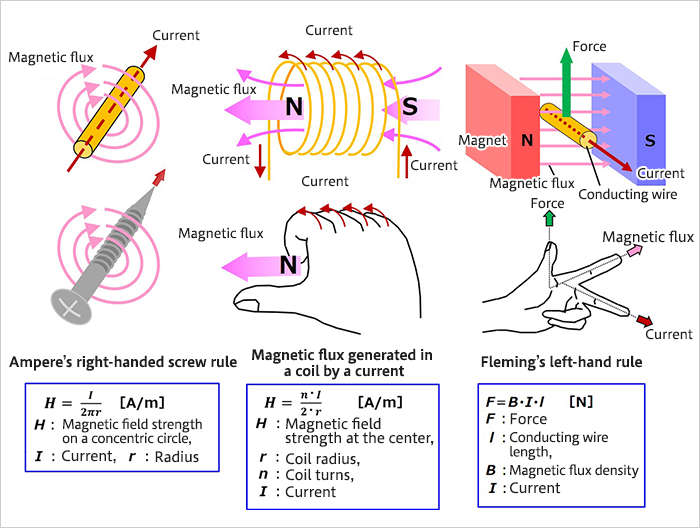## Principle of Motor Rotation

The principle of motor rotation is explained, using figures and equations.

When a conducting wire has a rectangular shape, the forces acting on the wire are considered.

The force F acting on the rectangle sides a and c is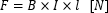As a result, a torque is generated about the central axis.

If for example we consider a state in which the rectangle has rotated by an angle θ, the force acting perpendicularly on b and d is the sin θ component, and so the torque Ta on the side a is represented by the following equation.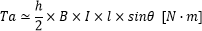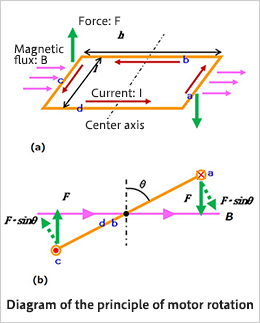Treating side c similarly, overall the torque is double, and so the following equation is used to calculate the torque generated.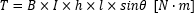The area of the rectangle is S＝h・l, and so substitution into the above equation yields the following.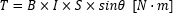This equation holds not only for a rectangle, but for circles and other shapes in general. The electric motor is a result of application of this principle.

#### Key Points:

・The principle of motor rotation is based on physical laws relating to currents, electric fields, and forces.